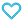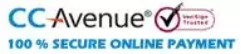# The Elements of Statics and Dynamics Part-2

95.00

The Elements of Statics and Dynamics Part-2 consist of brief theory of the chapter along with solved examples and practice exercises. It is useful for school and college students.

454 in stock

Qty:Add to WishlistMTG’s ORIGINAL MASPTERPIECE is a series of collection of books that started their journey as best sellers and continue as a chart-topper generation after generation. Even today these books are considered as a masterpiece among the teachers and students fraternity which is passionate about the subject.

The USP of MTG’s ORIGINAL MASTERPIECE Series lies in the fact that the work has been reproduced from the Original artifact and remains as true to the original work as possible.

The full solutions are provided to help students reaching the right answer systematically.

The student will find the book most useful if he uses it actively, that is to say, if he studies the relevant theoretical material carefully before going on to the worked-out solutions, and finally reinforces the newly-acquired knowledge by solving the problems given for independent work. The best results will be obtained when the student, having mastered the theoretical part, immediately attacks the unsolved problems without referring to the text solutions unless in difficulty.

• 1.  Velocity
•                Parallelogram of Velocities
•                 Relative Velocity
•                 Angular Velocity
• 2.   Acceleration..
•                 Parallelogram of Accelerations
•                 Relations between u, f, v, s, and t.
•                 Graphic Method
• 3.   Motion Under Gravity
•                 Galileo’s Experiment.
•                 Motion down a smooth inclined plane
•                 Lines of quickest descent
• 4.  The Laws of Motion
•                 The relation P = mf
•                 Physical Independence of Forces
•                 Parallelogram of Forces
• 5.  The Laws of Motion (Continued).
•           Application to Simple Problems
•                 Motion of two particles connected by a string.
•                 Motion on a rough inclined plane
•                 Atwood’s Machine..
• 6.  Impulse, Work and Energy.
•                 Motion of a shot and gun.
•                 Conservation of Energy
•                 Motion of the centre of inertia of a system of particles.
• 7.  Projectiles.
•                 Range on an inclined plane.
•                 Theoretical Proof that the path is a parabola.
•                 Experimental Proof.
• 8.  Collision of Elastic Bodies.
•                 Impact on a fixed plane.
•                 Direct impact of two spheres.
•                 Oblique impact of two spheres
•                 Loss of Kinetic Energy by Impact
• 9.  The Hodograph and Normal Accelerations
•                 The Conical Pendulum..
•                 Motion of a railway carriage on a curved portion
•                 of the railway line.
•                 Rotating sphere.
• 10. Motion on a Smooth Curve Under the Action of Gravity.
•                 Galileo’s Experiment.
•                 Motion on the outside of a vertical circle.
•                 Motion in a vertical circle.
•                 Newton’s Experimental Law.
• 11.  Simple Harmonic Motion, Pendulums.
•                 Time of oscillation of a simple pendulum.
•                 Experimental Verification.
•                 Determination of g by means of a simple pendulum.
• 12. Units and Dimensions.
•           Verification of formulae by means of counting the dimensions.
•           Table of Dimensions and Values of Fundamental
•           Quantities.
• 13.  Vectors.
•           Miscellaneous Examples.